Courses

Olympiad Test: Odd One Out - 2

20 Questions MCQ Test Mathematics Olympiad Class 7 | Olympiad Test: Odd One Out - 2

Description
Attempt Olympiad Test: Odd One Out - 2 | 20 questions in 40 minutes | Mock test for Class 7 preparation | Free important questions MCQ to study Mathematics Olympiad Class 7 for Class 7 Exam | Download free PDF with solutions
QUESTION: 1

Direction: In each problem, out of the five figures marked (1), (2), (3), (4) and (5), four are similar in a certain manner. However, one figure is not like the other four. Q. Choose the figure which is different from the rest.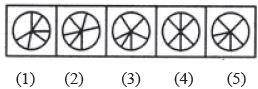Solution:

Only figure (4) contains three diameters of the circle.

QUESTION: 2

Direction: In each problem, out of the five figures marked (1), (2), (3), (4) and (5), four are similar in a certain manner. However, one figure is not like the other four. Q. Choose the figure which is different from the rest.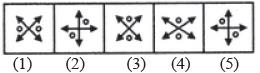Solution:

In all other figures, the two double sided arrows intersect each other at right angles.

QUESTION: 3

Direction: In each problem, out of the five figures marked (1), (2), (3), (4) and (5), four are similar in a certain manner. However, one figure is not like the other four. Q. Choose the figure which is different from the rest.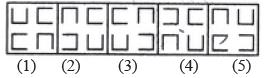Solution:

Only in figure (1), two of the four elements are oriented in the same direction.

QUESTION: 4

Direction: In each problem, out of the five figures marked (1), (2), (3), (4) and (5), four are similar in a certain manner. However, one figure is not like the other four.
Q. Choose the figure which is different from the rest.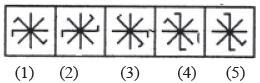Solution:

All other figures can be rotated into each other.

QUESTION: 5

Direction: In each problem, out of the five figures marked (1), (2), (3), (4) and (5), four are similar in a certain manner. However, one figure is not like the other four.
Q. Choose the figure which is different from the rest.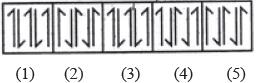Solution:

In all other figures, each element is obtained by rotating the adjacent element through 180°.

QUESTION: 6

Direction: In each problem, out of the five figures marked (1), (2), (3), (4) and (5), four are similar in a certain manner. However, one figure is not like the other four.
Q. Choose the figure which is different from the rest.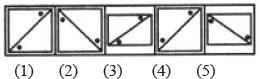Solution:

The two dots lie on the same side of the diagonal only in figure (4).

QUESTION: 7

Direction: In each problem, out of the five figures marked (1), (2), (3), (4) and (5), four are similar in a certain manner. However, one figure is not like the other four.
Q. Choose the figure which is different from the rest.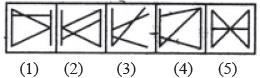Solution:

All other figures contain five line segments while figure (3) contains four line segments.

QUESTION: 8

Direction: In each problem, out of the five figures marked (1), (2), (3), (4) and (5), four are similar in a certain manner. However, one figure is not like the other four.
Q. Choose the figure which is different from the rest.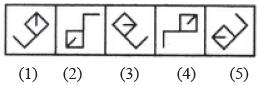Solution:

The line segment inside the square is attached to the corner which lies opposite to the corner to which the outer L-shaped element is attached.

QUESTION: 9

Direction: In each problem, out of the five figures marked (1), (2), (3), (4) and (5), four are similar in a certain manner. However, one figure is not like the other four.
Q. Choose the figure which is different from the rest.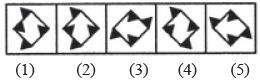Solution:

Only in figure (4), each side of the square has one black triangle attached to it.

QUESTION: 10

Direction: In each problem, out of the five figures marked (1), (2), (3), (4) and (5), four are similar in a certain manner. However, one figure is not like the other four.
Q. Choose the figure which is different from the rest.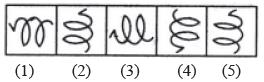Solution:

All other figures can be rotated into each other.

QUESTION: 11

Direction: In each problem, out of the five figures marked (1), (2), (3), (4) and (5), four are similar in a certain manner. However, one figure is not like the other four.
Q. Choose the figure which is different from the rest.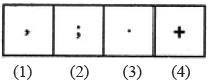Solution:

All others are punctuation marks.

QUESTION: 12

Direction: In each problem, out of the five figures marked (1), (2), (3), (4) and (5), four are similar in a certain manner. However, one figure is not like the other four.
Q. Choose the figure which is different from the rest.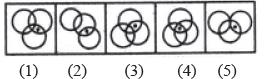Solution:

Only in figure (4), the dot appears in the region common to all the three circles.

QUESTION: 13

Direction: In each problem, out of the five figures marked (1), (2), (3), (4) and (5), four are similar in a certain manner. However, one figure is not like the other four.
Q. Choose the figure which is different from the rest.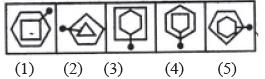Solution:

Only in figure (4), the pin passes through a vertex of each one of the two elements.

QUESTION: 14

Direction: In each problem, out of the five figures marked (1), (2), (3), (4) and (5), four are similar in a certain manner. However, one figure is not like the other four.
Q. Choose the figure which is different from the rest.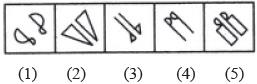Solution:

All other figures contain a geometrical figure along with its mirror image.

QUESTION: 15

Direction: In each problem, out of the five figures marked (1), (2), (3), (4) and (5), four are similar in a certain manner. However, one figure is not like the other four.
Q. Choose the figure which is different from the rest.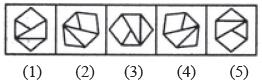Solution:

All other figures can be rotated into each other.

QUESTION: 16

Direction: In each problem, out of the five figures marked (1), (2), (3), (4) and (5), four are similar in a certain manner. However, one figure is not like the other four.
Q. Choose the figure which is different from the rest.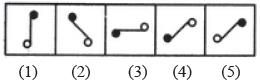Solution:

Figure (c) is different from other figures.

QUESTION: 17

Direction: In each problem, out of the five figures marked (1), (2), (3), (4) and (5), four are similar in a certain manner. However, one figure is not like the other four.
Q. Choose the figure which is different from the rest.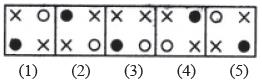Solution:

In each one of the figures except figure (3), the two crosses (×) appear in the diagonally opposite corners.

QUESTION: 18

Direction: In each problem, out of the five figures marked (1), (2), (3), (4) and (5), four are similar in a certain manner. However, one figure is not like the other four.
Q. Choose the figure which is different from the rest.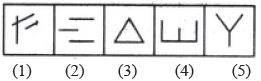Solution:

Figure (4) is formed by four line segments while each one of the other figures is formed by three line segments.

QUESTION: 19

Direction: In each problem, out of the five figures marked (1), (2), (3), (4) and (5), four are similar in a certain manner. However, one figure is not like the other four.
Q. Choose the figure which is different from the rest.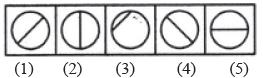Solution:

Only in figure (3), the line segment is not a diameter of the circle.

QUESTION: 20

Direction: In each problem, out of the five figures marked (1), (2), (3), (4) and (5), four are similar in a certain manner. However, one figure is not like the other four.
Q. Choose the figure which is different from the rest.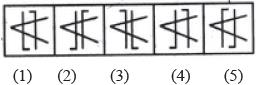Solution:

Only in figure (4), both the parallel lines are bent in the same direction {i.e. towards the left).Use Code STAYHOME200 and get INR 200 additional OFF Use Coupon Code

Track your progress, build streaks, highlight & save important lessons and more!

Similar ContentRelated tests